# Moment of inertia

## Definition

### For a single particle about an axis

The moment of inertia of a particle of mass$m$ about an axis$\ell$ is defined as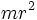$mr^2$, where$r$ is the perpendicular distance from$m$ to$\ell$.

### For a finite collection of particles about an axis

The moment of inertia about a system of particles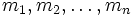$m_1, m_2, \dots, m_n$ about an axis$\ell$ is defined as:$\sum_{i=1}^n m_ir_i^2 = m_1r_1^2 + m_2r_2^2 + \dots + m_nr_n^2$

where$r_i$ is the perpendicular distance from$m_i$ to$\ell$.

### For a rigid body about an axis

The moment of inertia of a rigid body about an axis$\ell$ is defined as: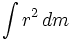$\int r^2 \, dm$

where, for a mass differential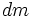$dm$ of the body,$r$ is defined as the perpendicular distance from the mass differential to$\ell$. This is equivalent to: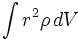$\int r^2 \rho \, dV$

where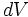$dV$ is the volume differential and$\rho$ is the density. For a body of constant density,$\rho$ can be pulled out of the integral, and we obtain:$\rho \int r^2 \, dV$

## Units and dimensions

Scalar or vector? Scalar
MLT dimensions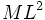$ML^2$: MLT;1;2;0
SI units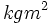$kgm^2$ (kilograms meter-squared)

## Main facts

There are two main facts used to compute moment of inertia:

## For typical shapes

Below is the moment of inertia of bodies of constant density and mass$m$ with some typical shapes. Note that for the first four of these shapes, all rotations about the specified axis are symmetries of the figure, so performing these rotations does not change the geometry in so far as contact with other surfaces is concerned:

Shape and parameters Axis Moment of inertia Radius of gyration Quotient$I/mr^2$ where$r$ is a natural radius of rotation about that axis
solid cylinder of base radius$r$ axis of cylinder, passes through centers of all the circles$(1/2)mr^2$$r/\sqrt{2}$ 1/2
open-ended hollow cylinder of base radius$r$ axis of cylinder, passes through centers of all the circles$mr^2$$r$ 1
solid sphere of radius$r$ any axis through the center of the sphere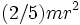$(2/5)mr^2$$r\sqrt{2/5}$ 2/5
hollow sphere of radius$r$ any axis through the center of the sphere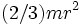$(2/3)mr^2$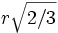$r\sqrt{2/3}$ 2/3
solid hemisphere of radius$r$ axis through the center perpendicular to the bounding circular disk$(2/5)mr^2$$r\sqrt{2/5}$ --
closed hollow hemisphere of radius$r$ axis through the center perpendicular to the bounding circular disk$(19/18)mr^2$$r\sqrt{19/18}$ --
solid right circular cone of base radius$r$, height$h$ axis through the vertex, along height, perpendicular to base$(3/10)mr^2$$r\sqrt{3/10}$ --
thin solid circular disk of radius$r$ axis through center, perpendicular to plane of circle$(1/2)mr^2$$r/\sqrt{2}$ 1/2
thin solid circular disk of radius$r$ axis through center, a diameter of circle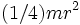$(1/4)mr^2$$r/2$ --
circular hoop (thin hollow circle) of radius$r$ axis through center, perpendicular to plane of circle$mr^2$$r$ 1
circular hoop (thin hollow circle) of radius$r$ axis through center, a diameter of circle$(1/2)mr^2$$r/\sqrt{2}$ --